Search IntMath
Close

450+ Math Lessons written by Math Professors and Teachers

5 Million+ Students Helped Each Year

1200+ Articles Written by Math Educators and Enthusiasts

Simplifying and Teaching Math for Over 23 Years

# The Equation of Time

By Murray Bourne, 26 Aug 2010

First, let's investigate the properties of an orbiting body.

In the following graph, the green ball represents the Earth rotating around the (red) Sun, which is at a focus of the ellipse.

This is greatly exaggerated so you can see what is going on.

Consider how fast the sun would appear to be moving when we are close to it, compared to when we are way out near the second focus of the ellipse (labeled F2.)


//<![CDATA[
var brd = JXG.JSXGraph.initBoard('jxgbox',{boundingbox:[-2.6,1.75,2.6,-1.5], keepaspectratio:true, showCopyright:false});
var p = brd.create('point',[0,1],{name:'Earth',face:'o', size:5, strokeColor:'green', fillColor:'green',fillOpacity:0.3, strokeOpacity: 0.3});
function plot(){
var a = 2.2;
var a2 = Math.pow(a,2);
var b = 1;
var b2 = Math.pow(b,2);
var c = Math.sqrt(Math.pow(a,2) - b2);
var f1 = brd.create('point',
[-c,0],
{name:"sun", face:'o', size:5, strokeColor:'red', fillColor:'red', fillOpacity:0.7, strokeOpacity: 0.7});
var f2 = brd.create('point',
[c,0],
{name:"F<sub>2</sub>",face:'o', size:1, strokeColor:'gray', fillColor:'gray', fillOpacity:0.3, strokeOpacity: 0.3});
var i = 0;
setInterval(function(){
if(Math.cos(i/100) < 0) {fudge=-1} else {fudge=1}
p.moveTo(
[a*Math.sin(i/100),
fudge*b*Math.sqrt(1-Math.pow(Math.sin(i/100),2))]
);
i++;
}
,10);
//brd.suspendUpdate();
}
plot();
copy = brd.create('text',[-2.4,-2,"Copyright &copy; <a href='https://www.intmath.com/'>www.intmath.com</a>"],{fontSize:12+'px'} );
//]]&gt;



[In fact, the Earth's orbit around the sun is very close to a circle, but it is still elliptical. See more on the Ellipse.]

## The Equation of Time

Many of us don't take much notice of the motion of the sun, moon or planets. (Let's face it - most of us live in places that have bad air and light pollution so we can hardly see the sky).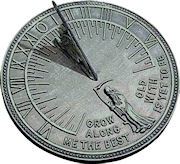Sundial [Image source]

Consequently, we assume the Earth's motion around the sun is uniform and that it takes exactly 24 hours for the sun to come back to the same spot above us each day.

But this is not so. There is as much as 30 minutes variation between the position of the sun relative to the stars at the same time of day, throughout the year. That is, the sun can be "behind" by as much as 14 min 6 sec (around 12 February each year) and up to 16 min 33 sec "ahead" (around 3rd Nov each year).

There are 2 reasons why this is so:

1. The Earth revolves around the Sun along an ellipse, not a circle. As we saw above, this means the Earth does not travel around the sun at a constant speed.
2. The Earth is tilted at 23.44 degrees

If the Earth rotated around the sun in a perfect circle and there was no tilt on the Earth's axis, we would see the sun overhead in exactly the same position every day.

The ancient Greeks knew about this issue but because they didn't have accurate clocks, they were not too concerned. By the 17th century, with the invention of pendulum clocks, knowing where the sun should be at any time of the year became critical for accuracy in navigation at sea.

## The Equation of Time

The Equation of Time takes the above 2 factors into consideration and can tell us how far ahead or behind the sun will be relative to the stars.

An explicit function that is a good approximation to the Equation of Time is as follows, where d is the day of the year and the resulting value is in terms of minutes of variation):

Time variation = -7.655 sin d + 9.873 sin(2d + 3.588)

This is made up of 2 components:

Variation due to Earth's tilt = -7.655 sin d

This contributes 7.655 minutes to the Equation of Time, and the period is 1 year (365.25 days).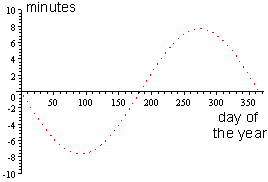This is also known as the Effect of Orbit Eccentricity. More strictly, adjusting for the correct period, its equation is: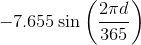The second component:

Variation due to elliptical orbit = 9.873 sin(2d + 3.588)

This contributes 9.873 minutes to the variation in the sun's position, and has period half of one year: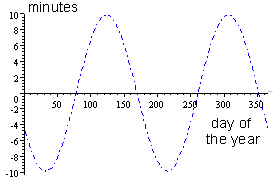This is also called the Effect of Obliquity. Once again, adjusting for the correct period, its equation more correctly is: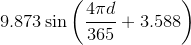[See more on Period of Trigonometric Graphs.]

When the 2 components are added together, we obtain: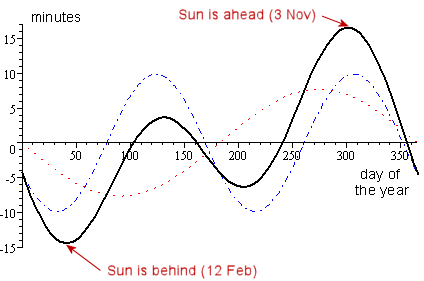Time variation = -7.655 sin d + 9.873 sin(2d + 3.588)

When we add ordinates of trigonometric functions like this, we obtain what is called a composite trigonometric curve. (See more on Composite Trigonometric Curves.)

(You can see how the Equation of Time is derived at Equation of Time.)

## Conclusion

The Equation of Time is a neat real-life application of conic sections (the Ellipse) and Composite Trigonometric Curves.

### 42 Comments on “The Equation of Time”

1. imran says:

no doubt u explain very nice , any one can uderstand it without hesitation

thanx
imran

2. Sihle says:

Thankyou for the helpful math newsleter you sent me plese sed me anything about the basics of calculus

3. Max Angell says:

I'm sorry to be the bearer of bad news, but your equation for the equation of time is slightly off. The 2nd component of the equation (the variation due to the elliptical orbit) needs to have a negative sign in front of it so that it reads:

-9.873*sin(2d + 3.588)

Therefore the total equations must read:

Time variation = -7.655*sin(d) - 9.873*sin(2d + 3.588)

Now if graphed, your equations would match the given graphs.

Thank you very much for the equations and the descriptions. I am teaching a trigonometry class and we are building a sundial for our final project and this site was very useful!

4. Max Angell says:

I actually just checked the calculations and I now notice that they must occur in degrees with a window of 2pi which then makes the current equations correct. Sorry for the false correction.

5. Murray says:

@Max: Thanks for the correction.

All the best with your sundial project!

6. Tom Moore says:

Your graphs for the separate components of the EOT are backwards. The upper graph is for the obliquity of the ecliptic (tilt) and the lower one is for the eccentricity of the earth's orbit. Not too serious but very confusing.

7. Murray says:

@Tom: Thanks for the feedback. You were right - the order should have been reversed for better clarity, so I did so. I also added some other information for better accuracy as regards the period.

8. Ricardo says:

Your formula has a maximum for d = 301, this coincides with October 28th. But this maximum has to be reached on November the 3th (or 4th)!
There seems to be a shift of a week!

9. Murray says:

The second formula has a maximum at 306.4 days, or Nov 2nd (or partway into the 3rd.)

10. Ricardo says:

Hi, Murray, thanks for your rapid response.
I've done the calculations several times, perhaps I'm not using the correct formula, but if I put
c = 2*PI/365.0
d = 301 (October 28th) in the formula
-7.655*sin(c*d) + 9.873*sin(2*c*d + 3.588)
then I get a value of
16.530433563385103

For d = 306 (November 2nd/3th) the value is 16.37737222674313
What am I doing wrong? Thanks for your patience!

11. Ricardo says:

Perhaps d is not the day of the year, but the number of days after the perihelion (4/5 January).

12. Alan Friot says:

Your depiction of how the two components contribute to the equation of time is incorrect. If you will send me an email address I will send you a paper explaining the new correct understanding.
Thank you
Alan friot

13. Murray says:

Here is the paper Alan sent me:

Equation of Time - Alan Friot

Thank you, Alan, for your insights.

14. Alan Friot says:

Will they now change the depiction of how the components contribute?

15. Murray says:

@Alan: I believe my article incorporates the conventional components for the equation of time.

Your paper introduces another perspective, so I included the link to it. Readers can decide which is the most appropriate one to follow.

16. Alan Friot says:

@Murray:
That's fine. By the way do you have any problem with my analysis? Both can not be correct.

17. Murray says:

As mentioned in my article, the components given are a "good approximation" for the equation/ Your final curve is roughly equivalent.

I'll try to have a closer look when I find some time, but in the meantime, I invite discussion from other readers.

18. Alan Friot says:

@Murray
That's fine, I assume you'll let me know the result of your review. No Rush! I have no question of the Equation of Time plot it is only the component curves that I believe are wrong.
Thanks
Alan

Hi, I was just wondering how you found the values for the two components for the equation of time. Mainly where the 9.873 and 3.588 came from in the variation due to elliptical orbit equation as well as the -7.655 in the variation due to the Earth's tilt. I'm curious because I'm trying to understand how those values would change, if this equation were to be calculated, for say a different planet. Thanks

20. Murray says:

@Maddie: The Wikipedia article on the topic has the derivation of the components. See: Calculating the Equation of Time

21. Alan Friot says:

I am afraid I'm no help to you. As I say below the figure, the ET plot has been made to scale. The curves for the contributors to the ET depict all that is currently known about then. Namely their general form and the times through out the year when they are at their maximum and minimum values.
Sorry
Alan

22. Murray says:

@Alan

Maddie's comment reminded me I needed to have a look at your analysis a bit more closely.

I checked your resulting curves once more and I don't believe they add together to give the Equation of Time.

I placed a grid behind your Figure 2 and added the ordinates of the two contributions (the reddish curve) and found the result was along way from the actual EOT curve.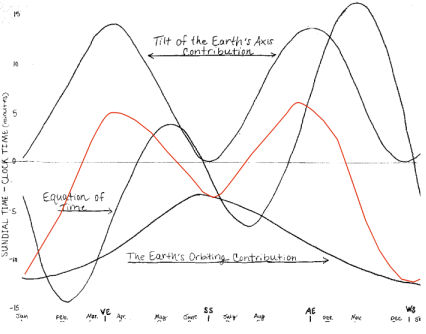Do you have equations for those curves?

23. Alan Friot says:

@Murry:
I did those curves at a dinning room table. My hands are not the most stable things so I actually had my grand daughter help me with them. They were meant to be representative not mathematical measuring tools. I do not have any equations for those curves. Anyone trying to establish meaningful equations should also make sure they are knowledgeable of my discovery and the correct value of the tip angle of the earth. To understand what I am saying go to "Alan,s Discovery' on you tube https://www.youtube.com/watch?v=EFlzzm_sE3Y .
What I explain can not be seen from a geocentric perspective! So astronomers and astrophysicists can not not comprehend what I am explaining.
Thanks
Alan

24. Murray says:

@Alan: I wasn't criticising your curve drawing at all! My point was that if your analysis concludes with those 2 curves, and they don't actually produce the Equation of time, then they are probably not the actual components.

25. Alan Friot says:

@Murray:
No one disagrees that those are the proper components. The manor the manor in which each contributes is were we differ. As you know my paper explains the new understanding which is done from a heliocentric perspective.

26. Alfred Bhulai says:

Hi,
How come our earliest sunset at 7 deg N. Lat. is on Nov 12?

27. Murray says:

@Alfred: To the nearest minute, the "peak" lasts for just over 2 weeks (as does the trough), so Nov 12th is within that peak period.

28. Chloe says:

Hi,
I was just wondering how you got the value of -3.588 on the second graph. I know that this is the horizontal translation, however I cannot understand how to get that value. Please explain.

29. Murray says:

@Chloe: If you observe the graph of that second component (the blue dotted curve), you'll notice it is a sine curve that has been shifted a certain amount. That is, it does not go through (0,0) but has been shifted to the right. See Graphs of y = a sin(bx + c) and y = a cos(bx + c) for more on phase shift.

30. Alan Friot says:

@Murray Do you agree with the fact that the earths orbiting the sun can only causes the time to get from one high noon to the next to increase? Compared to a fixed position of the earth with respect to the sun.

31. Alfred Bhulai says:

Murray,
No. I really do mean that the earliest sunset at 7 deg N. Lat. is November 12 -- to the nearest second! The softwares that calculate sunsets all show that the sun sets Nov 11, 12 & 13 at 17:31:31, but on Nov 10 at 17:31: 32, on Nov 14 at 17:31:33, and on Oct 31 at 17:32:36.

What I am after is an explanation or reference to one. Your answer suggests that you do not think your curves are that accurate; but when I plotted them on Mathcad I am definitely not getting the minimum on Nov 12.

This leads me to think that your curves are not valid for sunset times. I would dearly like to know what would do the trick. Would I need to use Bessel numbers for greater accuracy?

32. Murray says:

@Alan and Alfred: I was attracted to this topic because it's a nice application of composite trigonometric curves. I'm certainly no expert on where the actual equations come from nor can I vouch for their to-the-second accuracy.

Probably the best thing would be to approach the authors of the various papers referenced at the bottom of the Wikipedia article on the Equation of Time.

33. Alfred Bhulai says:

Thanks, Murray. I will do.

34. Winter Solstice | Waterford In Your Pocket says:

[…] The Winter Solstice is the shortest day of the year in terms of daylight, but it doesn’t have the latest sunrise or the earliest sunset of the year. This is because of a difference between our modern day timekeeping methods and howtime is measured using the Sun. Astronomers call this difference the equation of time. […]

35. Umma imon says:

E=59.7sin2l-98sinl-43.1cosl
is it right?

36. Murray says:

Is your question related to Equation of Time?

If not, the best place to ask it is in the

37. Laurence Graham says:

Murray,

Your article and graphs have helped me. Thank you. I am MOST impressed by your very kind and gentlemanly responses to all the comments you have received!

LG

38. christopher d parker says:

the earth is closest to the sun durring winter, movving throu angles very rapidly.SO!, I can now believe sun ries/set times on our our non-geometric clocks, could not coupe I checked this, and was astonished ! ...check this fact decades ago, could never get it.

39. Rob Gimberg says:

Is the equation of time a constant, irrespective of your Latitude or Longitude?

40. Murray says:

@Rob: The "equation" of time historically was an indication of the difference between what a sundial said the time was, and what the actual time should be, considering Earth's axis title and elliptic orbit.

The sundial was already designed for a particular latitude; the differences were applied to the time given by that actual sundial.

So in the sense you are asking, it is independent of latitude or longitude. (The differences were not quite constant because of year lengths being around 365.25 days, Earth's wobble and so on.)

You may be interested in this article which talks about it from the other way around: Applying the Equation of Time when calculating longitude at the Sun’s meridian Passage (Navigate without GPS)

41. Ron Hayward says:

Outstanding explanation. Straightforward, yet complete. Well done!

Thank you!

42. Winter Solstice | Waterford In Your Pocket says:

[…] The Winter Solstice is the shortest day of the year in terms of daylight, but it doesn’t have the latest sunrise or the earliest sunset of the year. This is because of a difference between our modern day timekeeping methods and how time is measured using the Sun. Astronomers call this difference the equation of time. […]

### Comment Preview

HTML: You can use simple tags like <b>, <a href="...">, etc.

To enter math, you can can either:

1. Use simple calculator-like input in the following format (surround your math in backticks, or qq on tablet or phone):
a^2 = sqrt(b^2 + c^2)
(See more on ASCIIMath syntax); or
2. Use simple LaTeX in the following format. Surround your math with $$ and $$.
$$\int g dx = \sqrt{\frac{a}{b}}$$
(This is standard simple LaTeX.)

NOTE: You can mix both types of math entry in your comment.